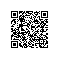# 贷还是不贷：如何用 Python 和机器学习帮你决策？## 任务• short_emp：一年以内短期雇佣

• emp_length_num：受雇年限

• home_ownership：居住状态（自有，按揭，租住）

• dti：贷款占收入比例

• purpose：贷款用途

• term：贷款周期

• last_delinq_none：贷款申请人是否有不良记录

• last_major_derog_none：贷款申请人是否有还款逾期90天以上记录

• revol_util：透支额度占信用比例

• total_rec_late_fee：逾期罚款总额

• safe_loans：贷款是否安全

## 决策“老张，吃了吗？”

……## 学习## 准备

http://t.cn/RoDJeNHpip install -U PIL

jupyter notebookJupyter Notebook已经正确运行。下面我们就可以正式编写代码了。

## 代码import pandas as pd

df = pd.read_csv('loans.csv')

df.head()df.shape

(46508, 13)

X = df.drop('safe_loans', axis=1)
y = df.safe_loans

X.shape

(46508, 12)

y.shape

(46508,)

X.head()from sklearn.preprocessing import LabelEncoder
from collections import defaultdict
d = defaultdict(LabelEncoder)
X_trans = X.apply(lambda x: d[x.name].fit_transform(x))
X_trans.head()from sklearn.cross_validation import train_test_split
X_train, X_test, y_train, y_test = train_test_split(X_trans, y, random_state=1)

X_train.shape

(34881, 12)

X_test.shape

(11627, 12)

from sklearn import tree
clf = tree.DecisionTreeClassifier(max_depth=3)
clf = clf.fit(X_train, y_train)

with open("safe-loans.dot", 'w') as f:
f = tree.export_graphviz(clf,
out_file=f,
max_depth = 3,
impurity = True,
feature_names = list(X_train),
class_names = ['not safe', 'safe'],
rounded = True,
filled= True )

from subprocess import check_call
check_call(['dot','-Tpng','safe-loans.dot','-o','safe-loans.png'])

from IPython.display import Image as PImage
from PIL import Image, ImageDraw, ImageFont
img = Image.open("safe-loans.png")
draw = ImageDraw.Draw(img)
img.save('output.png')
PImage("output.png")## 测试

test_rec = X_test.iloc[1,:]
clf.predict([test_rec])

array()

y_test.iloc

1

from sklearn.metrics import accuracy_score
accuracy_score(y_test, clf.predict(X_test))

0.61615205986066912

## 讨论

====================================分割线================================